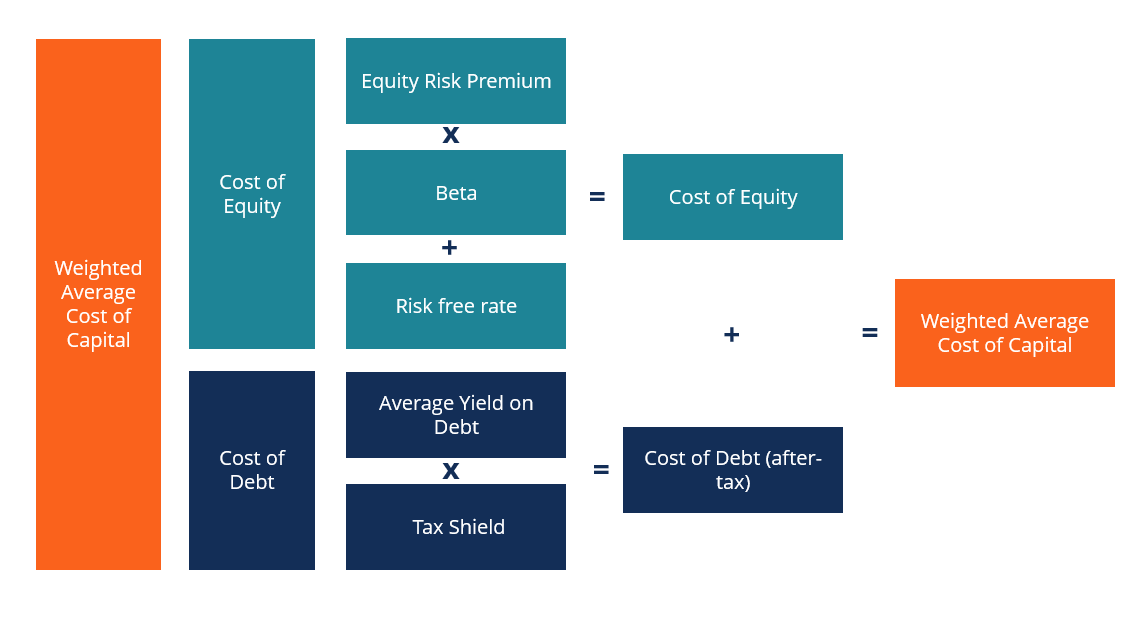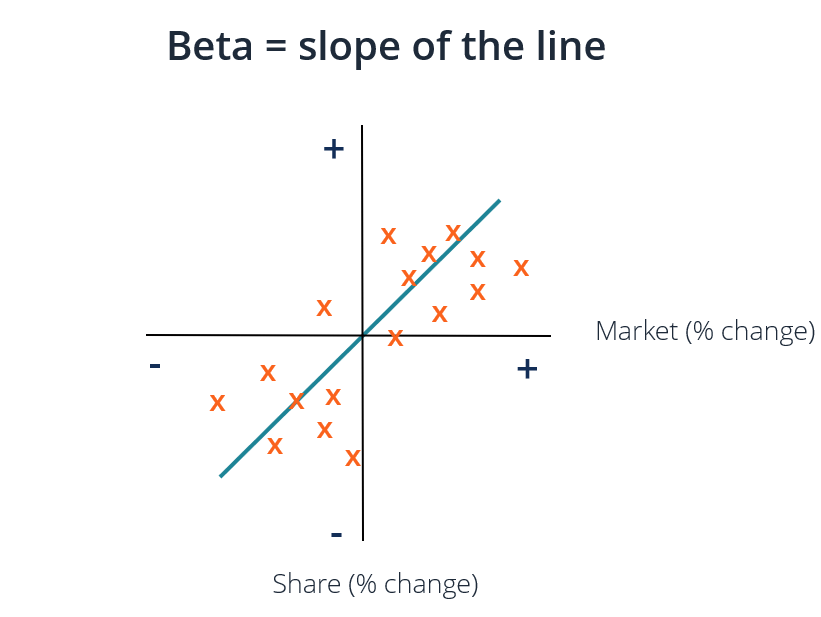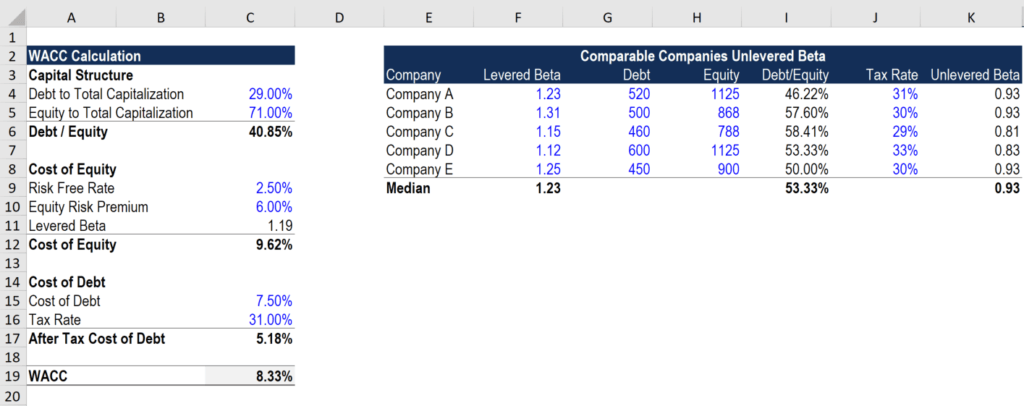How Can We Help?
Article of Contents

# Weighted Average Cost of Capital (WACC)

## Definition of WACC

A firm’s Weighted Average Cost of Capital (WACC) represents its blended cost of capital across all sources, including common shares, preferred shares, and debt.  The cost of each type of capital is weighted by its percentage of total capital and they are added together. This guide will provide a detailed breakdown of what WACC is, why it is used, how to calculate it, and will provide several examples.

WACC is used in financial modeling as the discount rate to calculate the net present value of a business.Image: CFI’s Business Valuation Modeling Course.

### What is the WACC Formula?

As shown below, the WACC formula is:

WACC  =  (E/V x Re)  +  ((D/V x Rd)  x  (1 – T))

Where:

E = market value of the firm’s equity (market cap)
D = market value of the firm’s debt
V = total value of capital (equity plus debt)
E/V = percentage of capital that is equity
D/V = percentage of capital that is debt
Re = cost of equity (required rate of return)
Rd = cost of debt (yield to maturity on existing debt)
T = tax rate

An extended version of the WACC formula is shown below, which includes the cost of Preferred Stock (for companies that have it).The purpose of WACC is to determine the cost of each part of the company’s capital structure based on the proportion of equity, debt, and preferred stock it has. Each component has a cost to the company. The company pays a fixed rate of interest on its debt and a fixed yield on its preferred stock. Even though a firm does not pay a fixed rate of return on common equity, it does often pay dividends in the form of cash to equity holders.

The weighted average cost of capital is an integral part of a DCF valuation model and, thus, it is an important concept to understand for finance professionals, especially for investment banking and corporate development roles. This article will go through each component of the WACC calculation.

### WACC Part 1 – Cost of Equity

The cost of equity is calculated using the Capital Asset Pricing Model (CAPM) which equates rates of return to volatility (risk vs reward).  Below is the formula for the cost of equity:

Re  =  Rf  +  β  ×  (Rm − Rf)

Where:

Rf = the risk-free rate (typically the 10-year U.S. Treasury bond yield)
β = equity beta (levered)
Rm = annual return of the market

The cost of equity is an implied cost or an opportunity cost of capital. It is the rate of return shareholders require, in theory, in order to compensate them for the risk of investing in the stock.  The Beta is a measure of a stock’s volatility of returns relative to the overall market (such as the S&P 500).  It can be calculated by downloading historical return data from Bloomberg or using the WACC and BETA functions.

#### Risk-free Rate

The risk-free rate is the return that can be earned by investing in a risk-free security, e.g., U.S. Treasury bonds. Typically, the yield of the 10-year U.S. Treasury is used for the risk-free rate.

Equity Risk Premium (ERP) is defined as the extra yield that can be earned over the risk-free rate by investing in the stock market. One simple way to estimate ERP is to subtract the risk-free return from the market return. This information will normally be enough for most basic financial analysis. However, in reality, estimating ERP can be a much more detailed task. Generally, banks take ERP from a publication called Ibbotson’s.

#### Levered Beta

Beta refers to the volatility or riskiness of a stock relative to all other stocks in the market. There are a couple of ways to estimate the beta of a stock. The first and simplest way is to calculate the company’s historical beta (using regression analysis) or just pick up the company’s regression beta from Bloomberg.

The second and more thorough approach is to make a new estimate for beta using public company comparables. To use this approach, the beta of comparable companies is taken from Bloomberg and the unlevered beta for each company is calculated.

Unlevered Beta = Levered Beta / ((1 + (1 – Tax Rate) * (Debt / Equity))

Levered beta includes both business risk and the risk that comes from taking on debt. However, since different firms have different capital structures, unlevered beta (asset beta) is calculated to remove additional risk from debt in order to view pure business risk. The average of the unlevered betas is then calculated and re-levered based on the capital structure of the company that is being valued.

Levered Beta = Unlevered Beta * ((1 + (1 – Tax Rate) * (Debt / Equity))

In most cases, the firm’s current capital structure is used when beta is re-levered. However, if there is information that the firm’s capital structure might change in the future, then beta would be re-levered using the firm’s target capital structure.

After calculating the risk-free rate, equity risk premium, and levered beta, the cost of equity = risk-free rate + equity risk premium * levered beta.Image: CFI’s Business Valuation Modeling Course.

### WACC Part 2 – Cost of Debt and Preferred Stock

Determining the cost of debt and preferred stock is probably the easiest part of the WACC calculation. The cost of debt is the yield to maturity on the firm’s debt and similarly, the cost of preferred stock is the yield on the company’s preferred stock. Simply multiply the cost of debt and the yield on preferred stock with the proportion of debt and preferred stock in a company’s capital structure, respectively.

Since interest payments are tax-deductible, the cost of debt needs to be multiplied by (1 – tax rate), which is referred to as the value of the tax shield. This is not done for preferred stock because preferred dividends are paid with after-tax profits.

Take the weighted average current yield to maturity of all outstanding debt then multiply it one minus the tax rate and you have the after-tax cost of debt to be used in the WACC formula.

Learn the details in CFI’s Math for Corporate Finance Course.

### WACC Calculator